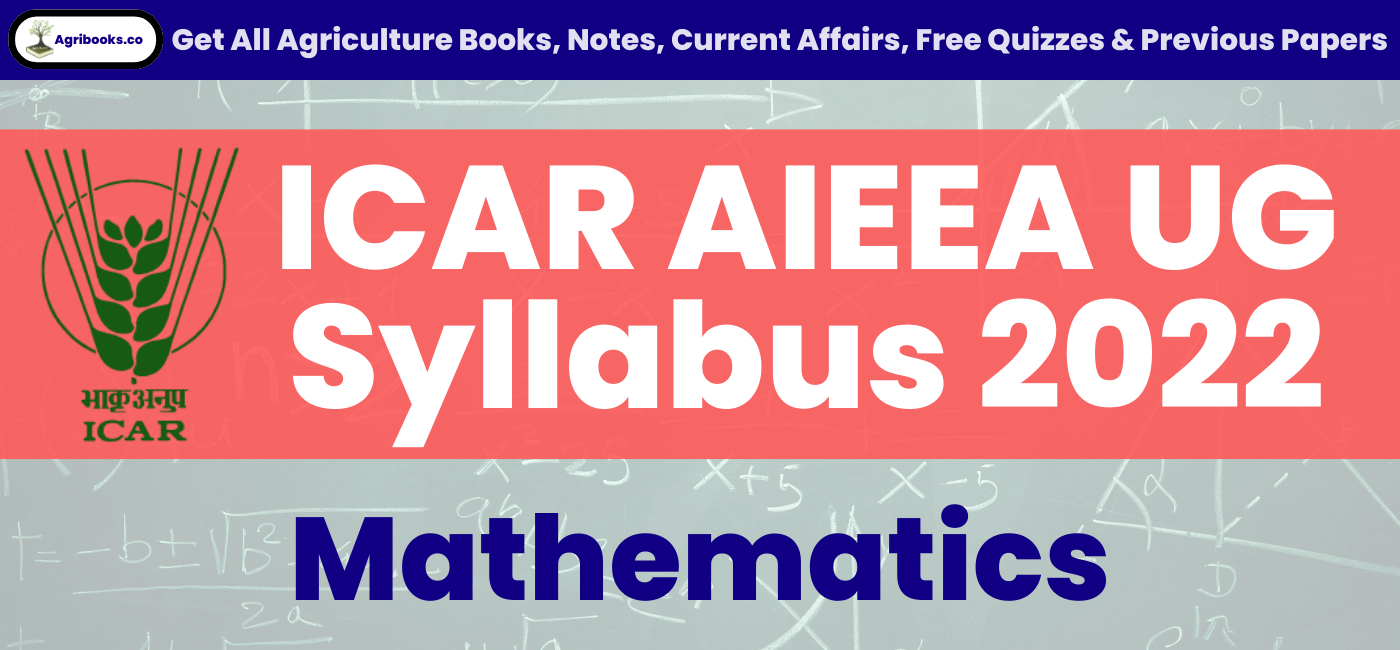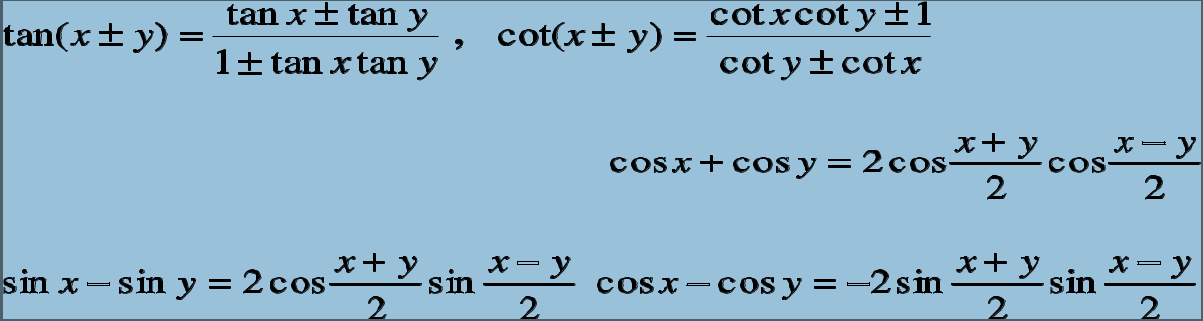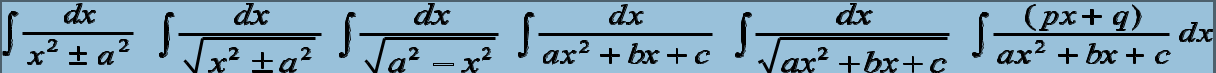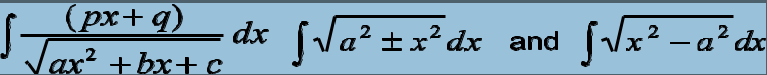###### ICAR AIEEA UG Syllabus 2022 for MathematicsStudents those are preparing for ICAR AIEEA UG Exams should know about the syllabus. We have mentioned syllabus of ICAR AIEEA UG Mathematics. Examination questions are comes from the syllabus so they should have syllabus.

Unit-1: Sets and Functions
1. Sets : Sets and their representations. Empty set. Finite & Infinite sets. Equal sets. Subsets, Subsets of the set of real numbers especially intervals (with notations). Power set. Universal set. Venn diagrams. Union and Intersection of sets. Difference of sets. Complement of a set.
2. Relations & Functions: Ordered pairs, Cartesian product of sets. Number of elements in the cartesian product of two finite sets. Cartesian product of the reals with itself (upto R x R x R). Definition of relation, Types of relations: reflexive, symmetric, transitive and equivalence relations. One to one and onto functions, composite functions, inverse of a function. Binary operations, Pictorial representation of a function, domain. Co-domain and range of a relation. Function as a special kind of relation from one set to
another. Real valued function of the real variable, domain and range of these functions, constant, identity, polynomial, rational, modulus, signum and greatest integer functions with their graphs. Sum, difference, product and quotients of functions.
3. Trigonometric Functions: Positive and negative angles. Measuring angles in radians & in degrees and conversion from one measure to another. Definition of trigonometric functions with the help of unit circle. Truth of the identity sin2x + cos2x=1, for all x. Signs of trigonometric functions and sketch of their graphs. Expressing sin (x+y) and cos (x+y) in terms of sinx, siny, cosx&cosy. Deducing the identities like the following: Identities related to sin2x, cos2x, tan2x, sin3x, cos3x and tan3x. General solution of trigonometric equations of the type sin è ?= sin á, cos è ?= cos á ?and tan è ?= tan á.
Inverse Trigonometric Functions: Definition, range, domain, principal value branches. Graphs of inverse trigonometric functions. Elementary properties of inverse trigonometric functions.
Properties of triangles, including centroid, incentre, circum-centre and orthocentre, Solution of triangles.
Heights and Distances.

Unit-2: Algebra
1. Principle of Mathematical Induction: Processes of the proof by induction, motivating the application of the method by looking at natural numbers as the least inductive subset of real numbers. The principle of mathematical induction and simple applications.
2. Complex Numbers and Quadratic Equations: Need for complex numbers, especially -1, to be motivated by inability to solve every quadratic equation. Brief description of algebraic properties of complex numbers. Argand plane and polar representation of complex numbers. Statement of Fundamental Theorem of Algebra, solution of quadratic equations in the complex number system.
3. Linear Inequalities: Linear inequalities. Algebraic solutions of linear inequalities in one variable and their representation on the number line. Graphical solution of linear inequalities in two variables. Solution of system of linear inequalities in two variables- graphically.
4. Permutations & Combinations: Fundamental principle of counting. Factorial n. (n!). Permutations and combinations, derivation of formulae and their connections, simple applications.
5. Binomial Theorem: History, statement and proof of the binomial theorem for positive integral indices.
Pascal’s triangle, General and middle term in binomial expansion, simple applications.
6. Sequence and Series: Sequence and Series. Arithmetic progression (A. P.). arithmetic mean (A.M.) Geometric progression (G.P.), general term of a G.P., sum of n terms of a G.P., geometric mean (G.M.), relation between A.M. and G.M. Sum to n terms of the special series Ón, Ón2 and Ón3.
7. Matrices: Concept, notation, order, equality, types of matrices, zero matrix, transpose of a matrix, symmetric and skew symmetric matrices. Addition, multiplication and scalar multiplication of matrices, simple properties of addition, multiplication and scalar multiplication. Non-commutativity of multiplication of matrices and existence of non-zero matrices whose product is the zero matrix (restrict to square matrices of order 2). Concept of elementary row and column operations. Invertible matrices and proof of the uniqueness of inverse, if it exists.
8. Determinants: Determinant of a square matrix (up to 3 x 3 matrices), properties of determinants, minors, cofactors and applications of determinants in finding the area of a triangle. Adjoint and inverse of a square matrix. Consistency, inconsistency and number of solutions of system of linear equations by examples, solving system of linear equations in two or three variables (having unique solution) using inverse of a matrix.

Unit-3: Coordinate Geometry
1. Straight Lines:Slope of a line and angle between two lines. Various forms of equations of a line: parallel to axes, point-slope form, slope-intercept form, two-point form, intercepts form and normal form. General equation of a line. Distance of a point from a line.
2. Conic Sections: Sections of a cone: circle, ellipse, parabola, hyperbola, a point, a straight line and pair of intersecting lines as a degenerated case of a conic section. Standard equations and simple properties of parabola, ellipse and hyperbola. Standard equation of a circle.
3. Introduction to Three-dimensional Geometry: Coordinate axes and coordinate planes in three dimensions. Coordinates of a point. Distance between two points and section formula.Unit-4: Calculus
1. Limits and Derivatives: Derivative introduced as rate of change both as that of distance function and geometrically, intuitive idea of limit. Definition of derivative, relate it to slope of tangent of the curve, derivative of sum, difference, product and quotient of functions. Derivatives of polynomial and trigonometric functions.
2. Continuity and Differentiability: Continuity and differentiability, derivative of composite functions, chain rule, derivatives of inverse trigonometric functions, derivative of implicit function. Concept of exponential and logarithmic functions and their derivative. Logarithmic differentiation. Derivative of functions expressed in parametric forms. Second order derivatives. Rolle’s and Lagrange’s Mean Value Theorems (without proof) and their geometric interpretations.
3. Applications of Derivatives: Applications of derivatives: rate of change, increasing/decreasing functions, tangents &normals, approximation, maxima and minima (first derivative test motivated geometrically and second derivative test given as a provable tool). Simple problems.
4. Integrals: Integration as inverse process of differentiation. Integration of a variety of functions by substitution, by partial fractions and by parts; only simple integrals of the type to be evaluated. Definite integrals as a limit of a sum, Fundamental Theorem of Calculus (without proof). Basic properties of definite integrals and evaluation of definite integrals.5. Applications of the Integrals: Applications in finding the area under simple curves, especially lines, areas of circles/ parabolas/ellipses (in standard form only), area between the two above said curves.
6. Differential Equations: Definition, order and degree, general and particular solutions of a differential equation. Formation of differential equation whose general solution is given. Solution of differential
equations by method of separation of variables, homogeneous differential equations of first order and first
degree. Solutions of linear
differential equation of the type: + = , where p and q are functions of x.

Unit-5: Vectors and Three-Dimensional Geometry
1. Vectors: Vectors and scalars, magnitude and direction of a vector. Direction cosines/ratios of vectors. Types of vectors (equal, unit, zero, parallel and collinear vectors), position vector of a point, negative of a vector, components of a vector, addition of vectors, multiplication of a vector by a scalar, position vector of a point dividing a line segment in a given ratio. Scalar (dot) product of vectors, projection of a vector on a line. Vector (cross) product of vectors.
2. Three-dimensional Geometry: Direction cosines/ratios of a line joining two points. Cartesian and vector equation of a line, coplanar and skew lines, shortest distance between two lines. Cartesian and vector equation of a plane. Angle between (i) two lines, (ii) two planes. (iii) a line and a plane. Distance of a point from a plane.

Unit-6: Linear Programming
Linear Programming: Introduction, definition of related terminology such as constraints, objective function, optimization, different types of linear programming (L.P.) problems, mathematical formulation of L.P.
problems, graphical method of solution for problems in two variables, feasible and infeasible regions, feasible and infeasible solutions, optimal feasible solutions (up to three non-trivial constraints).

Unit-7: Mathematical Reasoning
Mathematical Reasoning: Mathematically acceptable statements. Connecting words/ phrases – consolidating the understanding of “if and only if (necessary and sufficient) condition”, “implies”, “and/or”, “implied by”, “and”, “or”, “there exists” and their use through variety of examples related to real life and Mathematics. Validating the statements involving the connecting words, difference between contradiction, converse and contrapositive.

Unit-8: Statistics & Probability
1. Statistics: Measures of central tendency, mean, median and mode from ungrouped/grouped data. Measures of dispersion, mean deviation, variance and standard deviation from ungrouped/grouped data. Correlation, regression lines.
2. Probability: Random experiments: outcomes, sample spaces (set representation). Events: occurrence of events, ‘not’, ‘and’ and ‘or’ events, exhaustive events, mutually exclusive events Axiomatic (set theoretic) probability, Probability of an event, probability of ‘not’, ‘and’ & ‘or’ events. Multiplication theorem on probability. Conditional probability, independent events, total probability, Bayes’ theorem, Random variable and its probability distribution, mean and variance of stochastic variable. Repeated independent (Bernoulli) trials and Binomial distribution.Unit-9: Statics
Introduction, basic concepts and basic laws of mechanics, force, resultant of forces acting at a point, parallelogram law of forces, resolved parts of a force, Equilibrium of a particle under three concurrent forces. Triangle law of forces and its converse, Lami’s theorem and its converse, Two Parallel forces, like and unlike parallel forces, couple and its moment.

Unit-10: Dynamics
Speed and velocity, average speed, instantaneous speed, acceleration and retardation, resultant of two velocities. Motion of a particle along a line, moving with constant acceleration. Motion under gravity. Laws of motion, Projectile motion.

Share to Nearest and Dearest